# Floating Point Numbers5

Posted by Cleve Moler,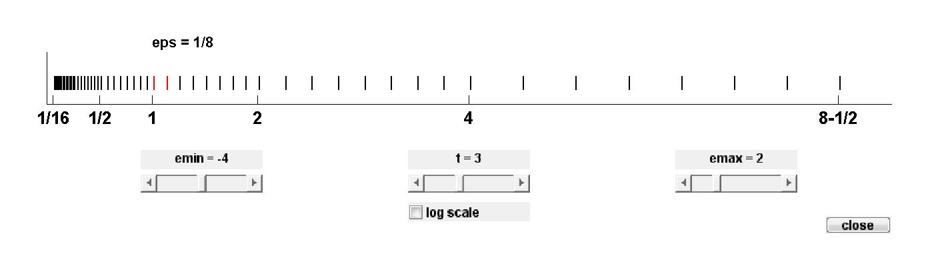This is the first part of a two-part series about the single- and double precision floating point numbers that MATLAB uses for almost all of its arithmetic operations. (This post is adapted from section 1.7 of my book Numerical Computing with MATLAB, published by MathWorks and SIAM.) ... read more >>

# Ordinary Differential Equations, Stiffness3

Posted by Cleve Moler,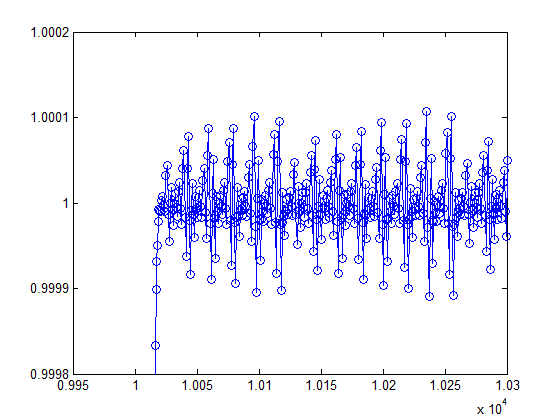# Ordinary Differential Equation Solvers ODE23 and ODE454

Posted by Cleve Moler,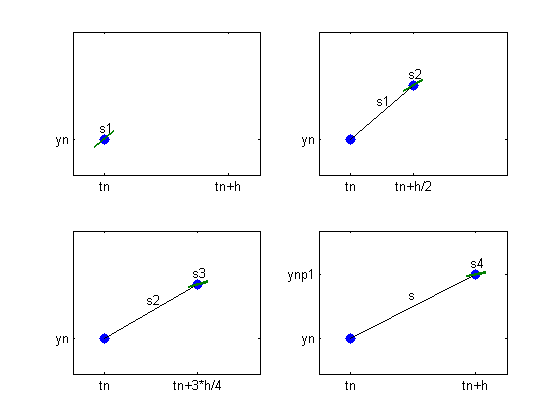The functions ode23 and ode45 are the principal MATLAB and Simulink tools for solving nonstiff ordinary differential equations.... read more >>

# Ordinary Differential Equation Suite4

Posted by Cleve Moler,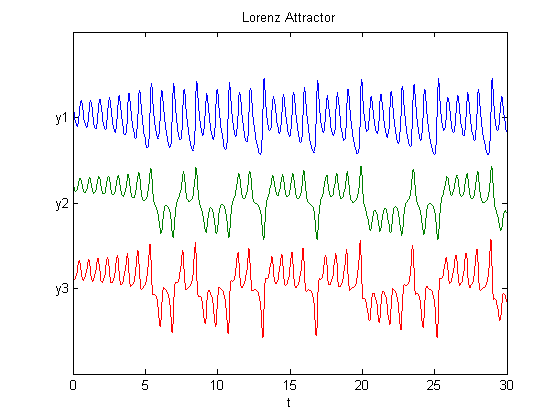MATLAB and Simulink have a powerful suite of routines for the numerical solution of ordinary differential equations. Today's post offers an introduction. Subsequent posts will examine several of the routines in more detail.... read more >>

# Fiedler Companion Matrix

Posted by Cleve Moler,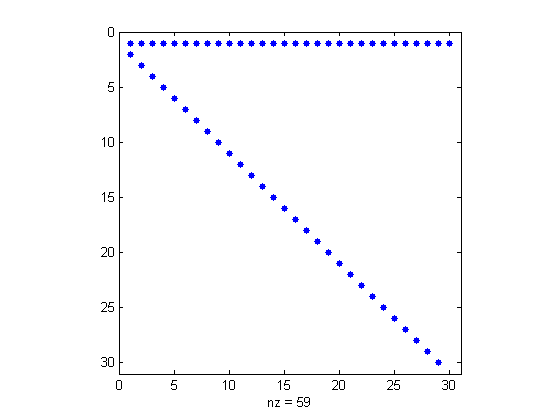The Fiedler companion matrix distributes the coefficients of a polynomial along the diagonals of an elegant pentadiagonal matrix whose eigenvalues are equal to the zeros of the polynomial.... read more >>

# Complex Step Differentiation3

Posted by Cleve Moler,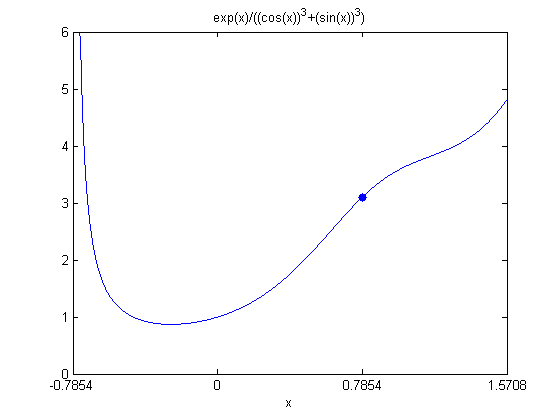Complex step differentiation is a technique that employs complex arithmetic to obtain the numerical value of the first derivative of a real valued analytic function of a real variable, avoiding the loss of precision inherent in traditional finite differences.... read more >>

# The Lambert W Function1

Posted by Cleve Moler,The Lambert W function deserves to be better known. It pops up in all sorts of places. And our MATLAB function for evaluating the function is a beautiful use of the Halley method.... read more >>